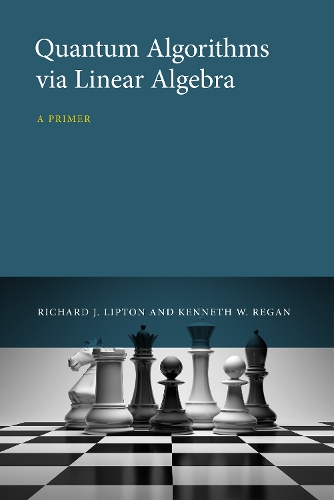•Quantum Algorithms via Linear Algebra: A Primer - The MIT Press (Hardback)

(author), (author)
£35.00
Hardback 206 Pages / Published: 05/12/2014
• We can order this

Usually dispatched within 1 week

Quantum computing explained in terms of elementary linear algebra, emphasizing computation and algorithms and requiring no background in physics. This introduction to quantum algorithms is concise but comprehensive, covering many key algorithms. It is mathematically rigorous but requires minimal background and assumes no knowledge of quantum theory or quantum mechanics. The book explains quantum computation in terms of elementary linear algebra; it assumes the reader will have some familiarity with vectors, matrices, and their basic properties, but offers a review of all the relevant material from linear algebra. By emphasizing computation and algorithms rather than physics, this primer makes quantum algorithms accessible to students and researchers in computer science without the complications of quantum mechanical notation, physical concepts, and philosophical issues. After explaining the development of quantum operations and computations based on linear algebra, the book presents the major quantum algorithms, from seminal algorithms by Deutsch, Jozsa, and Simon through Shor's and Grover's algorithms to recent quantum walks. It covers quantum gates, computational complexity, and some graph theory. Mathematical proofs are generally short and straightforward; quantum circuits and gates are used to illuminate linear algebra; and the discussion of complexity is anchored in computational problems rather than machine models. Quantum Algorithms via Linear Algebra is suitable for classroom use or as a reference for computer scientists and mathematicians.

Publisher: MIT Press Ltd
ISBN: 9780262028394
Number of pages: 206
Weight: 440 g
Dimensions: 229 x 152 x 17 mm

MEDIA REVIEWS
The book offers an easy innovative way to deal with quantum computation by the simple language of linear algebra and is highly recommended to anyone interested in quantum computation. -Zentralblatt MATH